# Multiband Dynamic Range Compression

This example shows how to simulate a digital audio multiband dynamic range compression system.

### Introduction

Dynamic range compression reduces the dynamic range of a signal by attenuating the level of strong peaks, while leaving weaker peaks unchanged. Compression has applications in audio recording, mixing, and in broadcasting.

Multiband compression compresses different audio frequency bands separately, by first splitting the audio signal into multiple bands and then passing each band through its own independently adjustable compressor. Multiband compression is widely used in audio mastering and is often included in audio workstations.

The multiband compressor in this example first splits an audio signal into different bands using a multiband crossover filter. Linkwitz-Riley crossover filters are used to obtain an overall allpass frequency response. Each band is then compressed using a separate dynamic range compressor. Key compressor characteristics, such as the compression ratio, the attack and release time, the threshold and the knee width, are independently tunable for each band. The effect of compression on the dynamic range of the signal is showcased.

A Linkwitz-Riley crossover filter consists of a combination of a lowpass and highpass filter, each formed by cascading two lowpass or highpass Butterworth filters. Summing the response of the two filters yields a gain of 0 dB at the crossover frequency, so that the crossover acts like an allpass filter (and therefore introducing no distortion in the audio signal).

`crossoverFilter` may be used to implement a Linkwitz-Riley System object. Since a Linkwitz-Riley crossover filter is formed by cascading two Butterworth filters, its order is always even. A Butterworth filter's slope is equal to 6*N dB/octave, where N is the filter order. When the `CrossoverSlopes` property of `crossoverFilter` is divisible by `12` (i.e. the filter is even-ordered), the object implements a Linkwitz-Riley crossover. Otherwise, the object implements a Butterworth crossover, where the lowpass and highpass sections are each implemented using a single Butterworth filter of order `CrossoverSlopes/6`.

Here is an example where an fourth-order Linkwitz-Riley crossover is used to filter a signal. Notice that the lowpass and highpass sections each have a -6 dB gain at the crossover frequency. The sum of the lowpass and highpass sections is allpass.

```Fs = 44100; % Linkwitz-Riley filter crossover = crossoverFilter(1,5000,4*6,Fs); % Transfer function estimator transferFuncEstimator = dsp.TransferFunctionEstimator( ... 'FrequencyRange','onesided','SpectralAverages',20); frameLength = 1024; scope = dsp.ArrayPlot( ... 'PlotType','Line', ... 'YLimits',[-40 1], ... 'YLabel','Magnitude (dB)', ... 'XScale','log', ... 'SampleIncrement',(Fs/2)/(frameLength/2+1), ... 'XLabel','Frequency (Hz)', ... 'Title','Eighth order Linkwitz-Riley Crossover Filter', ... 'ShowLegend',true, ... 'ChannelNames',{'Band 1','Band 2','Sum'}); tic while toc < 10 in = randn(frameLength,1); % Return lowpass and highpass responses of the crossover filter [ylp,yhp] = crossover(in); % sum the responses y = ylp + yhp; v = transferFuncEstimator(repmat(in,1,3),[ylp yhp y]); scope(20*log10(abs(v))); end```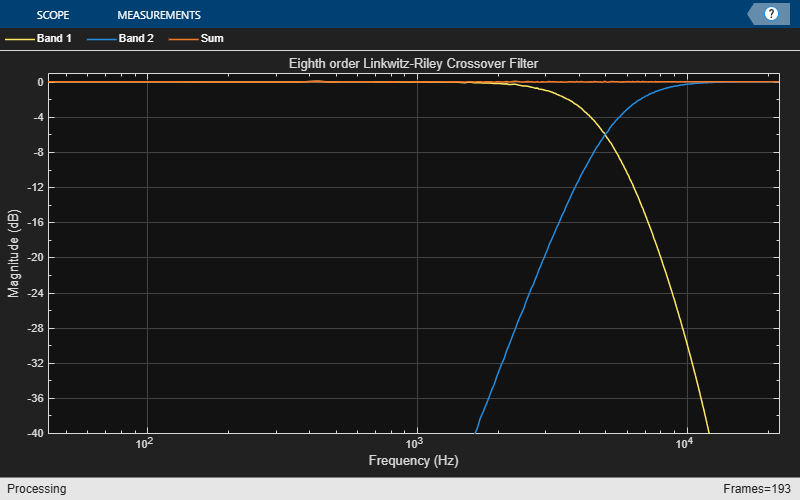### Multiband Crossover Filters

`crossoverFilter` may also be used to implement a multiband crossover filter by combining two-band crossover filters and allpass filters in a tree-like structure. The filter divides the spectrum into multiple bands such that their sum is a perfect allpass filter.

The example below shows a four-band crossover filter formed of fourth order Linkwitz-Riley crossover filters. Notice the allpass response of the sum of the four bands.

```Fs = 44100; crossover = crossoverFilter(3,[2e3 5e3 10e3],[24 24 24],44100); transferFuncEstimator = dsp.TransferFunctionEstimator('FrequencyRange','onesided','SpectralAverages',20); L = 2^14; scope = dsp.ArrayPlot( ... 'PlotType','Line', ... 'XOffset',0, ... 'YLimits',[-120 5], ... 'XScale','log', ... 'SampleIncrement', .5 * Fs/(L/2 + 1 ), ... 'YLabel','Frequency Response (dB)', ... 'XLabel','Frequency (Hz)', ... 'Title','Four-Band Crossover Filter', ... 'ShowLegend',true, ... 'ChannelNames',{'Band 1','Band 2','Band 3','Band 4','Sum'}); tic; while toc < 10 in = randn(L,1); % Split the signal into four bands [ylp,ybp1,ybp2,yhp] = crossover(in); y = ylp + ybp1 + ybp2 + yhp; z = transferFuncEstimator(repmat(in,1,5),[ylp,ybp1,ybp2,yhp,y]); scope(20*log10(abs(z))) end```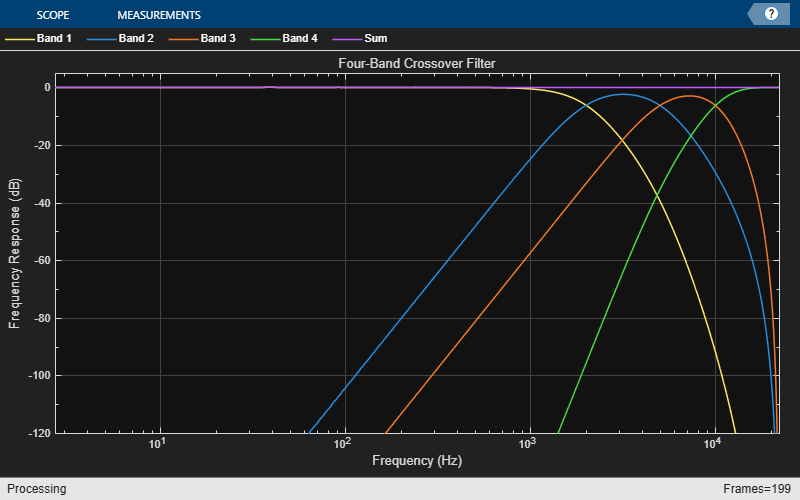### Dynamic Range Compression

`compressor` is a dynamic range compressor System object. The input signal is compressed when it exceeds the specified threshold. The amount of compression is controlled by the specified compression ratio. The attack and release times determine how quickly the compressor starts or stops compressing. The knee width provides a smooth transition for the compressor gain around the threshold. Finally, a make-up gain can be applied at the output of the compressor. This make-up gain amplifies both strong and weak peaks equally.

The static compression characteristic of the compressor depends on the compression ratio, the threshold and the knee width. The example below illustrates the static compression characteristic for a hard knee:

```drc = compressor(-15,5); visualize(drc); ```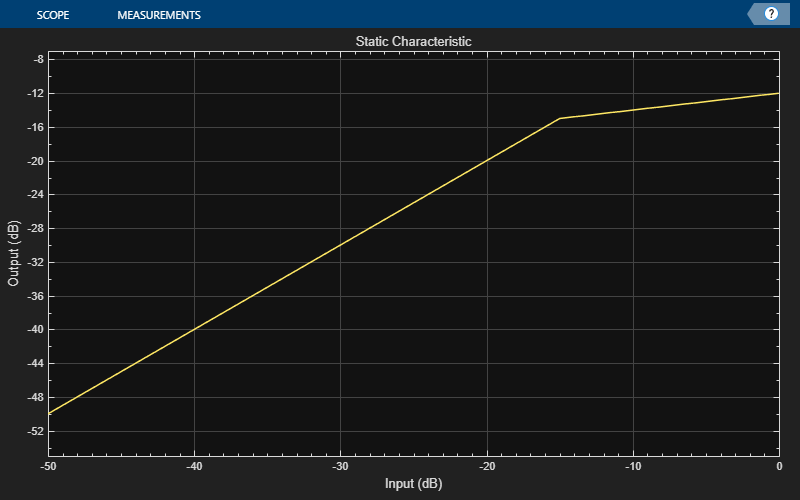In order to view the effect of threshold, ratio and knee width on the compressor's static characteristic, change the values of the `Threshold`, `Ratio` and `KneeWidth` properties. The static characteristic plot should change accordingly.

The compressor's attack time is defined as the time (in msec) it takes for the compressor's gain to rise from 10% to 90% of its final value when the signal level exceeds the threshold. The compressor's release time is defined as the time (in seconds) it takes the compressor's gain to drop from 90% to 10% of its value when the signal level drops below the threshold. The example below illustrates the signal envelope for different release and attack times:

```Fs = 44100; drc = compressor(-10,5, ... 'SampleRate',Fs, ... 'AttackTime',0.050, ... 'ReleaseTime',0.200, ... 'MakeUpGainMode','Property'); x = [ones(Fs,1);0.1*ones(Fs,1)]; [y,g] = drc(x); t = (1/Fs) * (0: 2*Fs - 1); figure subplot(211) plot(t,x); hold on grid on plot(t,y,'r') ylabel('Amplitude') legend('Input','Compressed Output') subplot(212) plot(t,g) grid on legend('Compressor gain (dB)') xlabel('Time (sec)') ylabel('Gain (dB)')```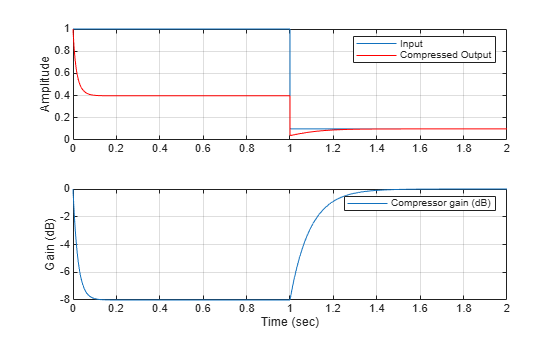The input maximum level is 0 dB, which is above the specified -10 dB threshold. The steady-state compressor output for a 0 dB input is -10 + 10/5 = -8 dB. The gain is therefore -8 dB. The attack time is defined as the time it takes the compressor gain to rise from 10% to 90% of its final value when the input level goes above the threshold, or in this case, from -0.8 dB to -7.2 dB. Let's find the times at which the gains in the compression stage are equal to -0.8 dB and -7.2 dB, respectively:

```[~,t1] = min(abs(g(1:Fs) + 0.1 * 8)); [~,t2] = min(abs(g(1:Fs) + 0.9 * 8)); tAttack = (t2 - t1) / Fs; fprintf('Attack time is %d s\n',tAttack)```
```Attack time is 5.000000e-02 s ```

The input signal then drops back down to 0, where there is no compression. The release time is defined as the time it takes the gain to drop from 90% to 10% of its absolute value when the input goes below the threshold, or in this case, -7.2 dB to -0.8 dB. Let's find the times at which the gains in the no-compression stage are equal to -7.2 dB and -0.8 dB, respectively:

```[~,t1] = min(abs(g(Fs:end) + 0.9 * 8)); [~,t2] = min(abs(g(Fs:end) + 0.1 * 8)); tRelease = (t2 - t1) / Fs; fprintf('Release time is %d s\n',tRelease)```
```Release time is 2.000000e-01 s ```

The example below illustrates the effect of dynamic range compression on an audio signal. The compression threshold is set to -15 dB, and the compression ratio is 7.

```frameLength = 1024; reader = dsp.AudioFileReader('Filename', ... 'RockGuitar-16-44p1-stereo-72secs.wav', ... 'SamplesPerFrame',frameLength); % Compressor. Threshold = -15 dB, ratio = 7 drc = compressor(-15,7, ... 'SampleRate',reader.SampleRate, ... 'MakeUpGainMode','Property', ... 'KneeWidth',5); scope = dsp.TimeScope('SampleRate',reader.SampleRate, ... 'TimeSpan',1,'BufferLength',Fs*4, ... 'ShowGrid',true, ... 'LayoutDimensions',[2 1], ... 'NumInputPorts',2, ... 'TimeSpanOverrunAction','Scroll'); scope.ActiveDisplay = 1; scope.YLimits = [-1 1]; scope.ShowLegend = true; scope.ChannelNames = {'Original versus compressed audio'}; scope.ActiveDisplay = 2; scope.YLimits = [-6 0]; scope.YLabel = 'Gain (dB)'; scope.ShowLegend = true; scope.ChannelNames = {'compressor gain in dB'}; while ~isDone(reader) x = reader(); [y,g] = drc(x); x1 = x(:,1); y1 = y(:,1); scope([x1,y1],g(:,1)) end```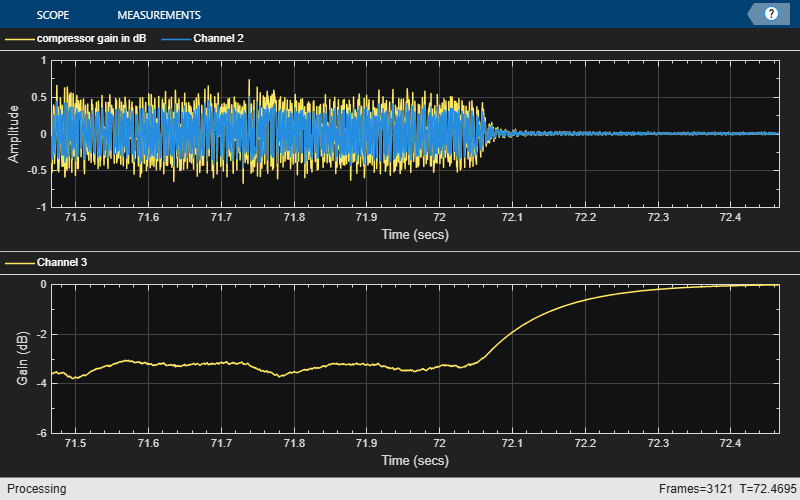### Simulink Version of the Multiband Dynamic Range Compression Example

The following model implements the multiband dynamic range compression example:

```model = 'audiomultibanddynamiccompression'; open_system(model)```

In this example, the audio signal is first divided into four bands using a multiband crossover filter. Each band is compressed using a separate compressor. The four bands are then recombined to form the audio output. The dynamic range of the uncompressed and compressed signals (defined as the ratio of the largest absolute value of the signal to the signal RMS) is computed. To hear the difference between the original and compressed audio signals, toggle the switch on the top level.

The model integrates a User Interface (UI) designed to interact with the simulation. The UI allows you to tune parameters and the results are reflected in the simulation instantly. To launch the UI that controls the simulation, click the 'Launch Parameter Tuning UI' link on the model.

```set_param(model,'StopTime','(1/44100) * 8192 * 20'); sim(model);```

Close the model:

`bdclose(model)`

### MATLAB Version of the Multiband Dynamic Range Compression Example

`HelperMultibandCompressionSim` is the MATLAB function containing the multiband dynamic range compression example's implementation. It instantiates, initializes and steps through the objects forming the algorithm.

The function `multibandAudioCompressionExampleApp` wraps around `HelperMultibandCompressionSim` and iteratively calls it. It also plots the uncompressed versus compressed audio signals. Plotting occurs when the `plotResults` input to the function is 'true'.

Execute `multibandAudioCompressionExampleApp` to run the simulation and plot the results on scopes. Note that the simulation runs for as long as the user does not explicitly stop it.

`multibandAudioCompressionExampleApp` launches a UI designed to interact with the simulation. The UI allows you to tune parameters and the results are reflected in the simulation instantly. For more information on the UI, please refer to `HelperCreateParamTuningUI`.

MATLAB Coder can be used to generate C code for the function `HelperMultibandCompressionSim`. In order to generate a MEX-file for your platform, execute `HelperMultibandCompressionCodeGeneration`.

By calling the wrapper function `multibandAudioCompressionExampleApp` with `'true'` as an argument, the generated MEX-file can be used instead of `HelperMultibandCompressionSim` for the simulation. In this scenario, the UI is still running inside the MATLAB environment, but the main processing algorithm is being performed by a MEX-file. Performance is improved in this mode without compromising the ability to tune parameters.

Call `multibandAudioCompressionExampleApp(true)` to use the MEX-file for simulation. Again, the simulation runs till the user explicitly stops it from the UI.

##### SupportGet trial now